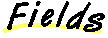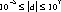`Packages  This Package  Prev  Next  Index  `

# §1.6 Class Double

```public  final  class  java.lang.Double
extends  java.lang.Number  (I-§1.11)
{
// Fields
public final static double MAX_VALUE;	§1.6.1
public final static double MIN_VALUE;	§1.6.2
public final static double NaN;	§1.6.3
public final static double NEGATIVE_INFINITY;	§1.6.4
public final static double POSITIVE_INFINITY;	§1.6.5

// Constructors
public Double(double  value);	§1.6.6
public Double(String  s);	§1.6.7

// Methods
public static long doubleToLongBits(double  value);	§1.6.8
public double doubleValue();	§1.6.9
public boolean equals(Object  obj);	§1.6.10
public float floatValue();	§1.6.11
public int hashCode();	§1.6.12
public int intValue();	§1.6.13
public boolean isInfinite();	§1.6.14
public static boolean isInfinite(double  v);	§1.6.15
public boolean isNaN();	§1.6.16
public static boolean isNaN(double  v);	§1.6.17
public static double longBitsToDouble(long  bits);	§1.6.18
public long longValue();	§1.6.19
public String toString();	§1.6.20
public static String toString(double  d);	§1.6.21
public static Double valueOf(String  s);	§1.6.22
}
```
This class wraps a value of the primitive type double in an object. An object of type Double contains a single field whose type is double.

In addition, this class provides a number of methods for converting a double to a String and a String to a double, as well as other constants and methods useful when dealing with a double.

##### MAX_VALUE

```public final static double ``` ``` MAX_VALUE = 1.79769313486231570e+308d ```
The largest positive value of type double.

### MIN_VALUE

```public final static double ``` ``` MIN_VALUE = 4.94065645841246544e-324 ```
The smallest positive finite value of type double.

### NaN

```public final static double NaN = 0.0 / 0.0 ```
A Not-a-Number value of type double.

### NEGATIVE_INFINITY

```public final static double ``` ``` NEGATIVE_INFINITY = -1.0 / 0.0 ```
The negative infinity of type double.

### POSITIVE_INFINITY

```public final static double ``` ``` POSITIVE_INFINITY = 1.0 / 0.0 ```
The positive infinity of type double.

##### Double

```public Double(double value) ```
Constructs a newly allocated Double object that represents the primitive double argument.
Parameters:
```value - ```the value to be represented by the Double

### Double

```public Double(String s) ``` ```throws NumberFormatException ```
Constructs a newly allocated Double object that represents the floating- point value of type double represented by the string. The string is converted to a double value as if by the valueOf method (I-§1.6.22).

Parameters:
```s - ```a string to be converted to a Double
Throws
NumberFormatException (I-§1.41)
If the string does not contain a parsable number.

##### doubleToLongBits

```public static long doubleToLongBits(double value) ```
The result is a representation of the floating-point argument according to the IEEE 754 floating-point "double format" bit layout.
Bit 63 represents the sign of the floating-point number. Bits 62-52 represent the exponent. Bits 51-0 represent the significand (sometimes called the mantissa) of the floating-point number.
If the argument is positive infinity, the result is 0x7ff0000000000000L.
If the argument is negative infinity, the result is 0xfff0000000000000L.
If the argument is NaN, the result is 0x7ff8000000000000L.
Parameters:
```value - ```a double precision floating point number
Returns:
the bits that represent the floating point number.

### doubleValue

```public double doubleValue() ```
Returns:
the double value represented by this object.
Overrides:
doubleValue in class Number (I-§1.11.1).

### equals

```public boolean equals(Object obj) ```
The result is true if and only if the argument is not null and is a Double object that represents a double that has the identical bit pattern to the bit pattern of the double represented by this object.
Note that in most cases, for two instances of class Double, d1 and d2, the value of d1.equals(d2) is true if and only if
d1.doubleValue() == d2.longValue()
also has the value true. However, there are two exceptions:
Parameters:
```obj - ```the object to compare with
Returns:
true if the objects are the same; false otherwise.
Overrides:
equals in class Object (I-§1.12.3).

### floatValue

```public float floatValue() ```
Returns:
the double value represented by this object is converted to type float and the result of the conversion is returned.
Overrides:
floatValue in class Number (I-§1.11.2).

### hashCode

```public int hashCode() ```
Returns:
a hash code value for this object.
Overrides:
hashCode in class Object (I-§1.12.6).

### intValue

```public int intValue() ```
Returns:
the double value represented by this object is converted to type int and the result of the conversion is returned.
Overrides:
intValue in class Number (I-§1.11.3).

### isInfinite

```public boolean isInfinite() ```
Returns:
true if the value represented by this object is positive infinity (I-§1.6.5) or negative infinity (I-§1.6.4); false otherwise.

### isInfinite

```public static boolean isInfinite(double v) ```
Parameters:
```v - ```the value to be tested
Returns:
true if the value of the argument is positive infinity (I-§1.6.5) or negative infinity (I-§1.6.4); false otherwise.

### isNaN

```public boolean isNaN() ```
Returns:
true if the value represented by this object is NaN (I-§1.6.3); false otherwise.

### isNaN

```public static boolean isNaN(double v) ```
Parameters:
```v - ```the value to be tested
Returns:
true if the value of the argument is NaN (I-§1.6.3); false otherwise.

### longBitsToDouble

```public static double longBitsToDouble(long bits) ```
The argument is considered to be a representation of a floating-point value according to the IEEE 754 floating-point "double precision" bit layout. That floating-point value is returned as the result.
If the argument is 0x7f80000000000000L, the result is positive infinity.
If the argument is 0xff80000000000000L, the result is negative infinity.
If the argument is any value in the range 0x7ff0000000000001L through 0x7fffffffffffffffL or in the range 0xfff0000000000001L through 0xffffffffffffffffL, the result is NaN. All IEEE 754 NaN values are, in effect, lumped together by the Java language into a single value.
Parameters:
```bits - ```any long integer
Returns:
the double floating-point value with the same bit pattern.

### longValue

```public long longValue() ```
Returns:
the double value represented by this object is converted to type long and the result of the conversion is returned.
Overrides:
longValue in class Number (I-§1.11.4).

### toString

```public String toString() ```
The primitive double value represented by this object is converted to a string exactly as if by the method toString of one argument (I-§1.6.21).

Returns:
a String representation of this object.
Overrides:
toString in class Object (I-§1.12.9).

### toString

```public static String toString(double d) ```
Creates a string representation of the double argument.
The values NaN, NEGATIVE_INFINITY, POSITIVE_INFINITY, -0.0, and +0.0 are represented by the strings "NaN", "-Infinity", "Infinity", "-0.0" and "0.0", respectively.
If d is in the range, then it is converted to a string in the style [-]ddd.ddd. Otherwise, it is converted to a string in the style [-]m.ddddE±±±±xx.
Warning to editor: I want a plus/minus symbol before the xx in the previous line. For some reason, some printers aren't printing itit. I don't completely understand
There is always a minimum of one digit after the decimal point. The number of digits is the minimum needed to uniquely distinguish the argument value from adjacent values of type double.
Parameters:
```d - ```the double to be converted
Returns:
a string representation of the argument.

### valueOf

```public static Double valueOf(String s) ``` ```throws NumberFormatException ```
Parses a string into a Double.
Parameters:
```s - ```the string to be parsed
Returns:
a newly constructed Double initialized to the value represented by the string argument..
Throws
NumberFormatException (I-§1.41)
If the string does not contain a parsable number.

`Packages  This Package  Prev  Next  Index`
Java API Document (HTML generated by dkramer on April 22, 1996)